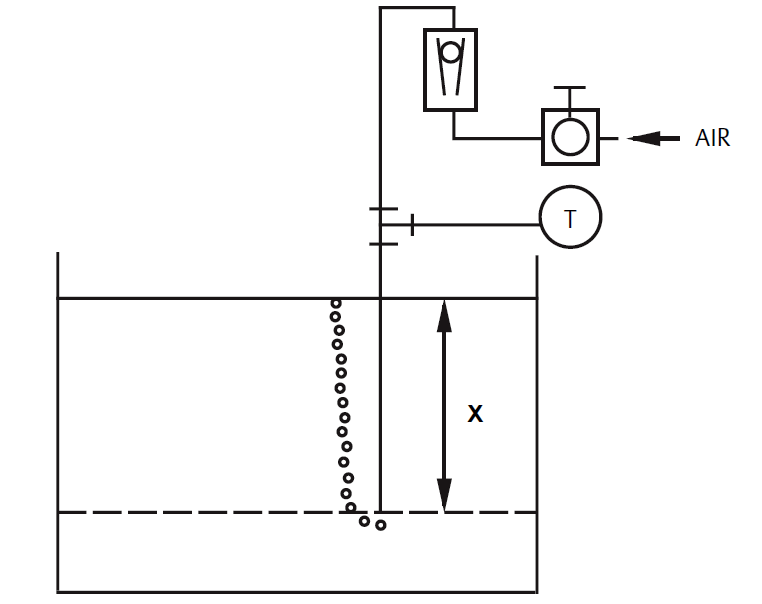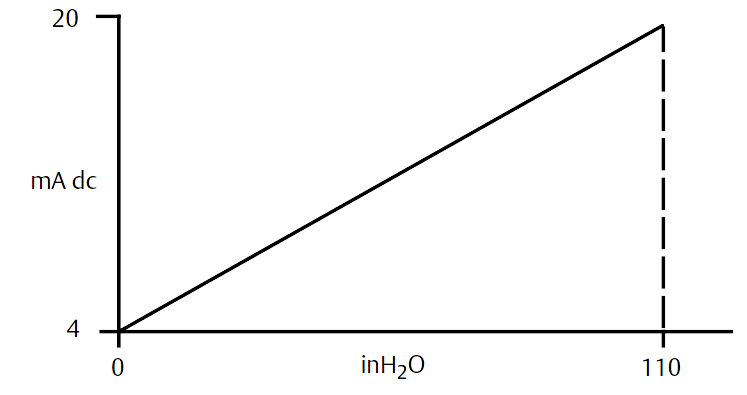# Bubbler Liquid Level Transmitter Calculation

Bubbler Liquid Level Transmitter CalculationImage Credits : Rosemount

Let X equal the vertical distance between the minimum and maximum measurable levels (100 in.).

Let SG equal the specific gravity of the fluid (1.1).

Let h equal the maximum head pressure to be measured in inches of water.

Let Range equal zero to h.

Then

h = (X)(SG)
= 100 x 1.1
= 110 inH2O

Range = 0 to 110 inH2OSource  : Rosemount

Don't Miss Our Updates
Be the first to get exclusive content straight to your email.
We promise not to spam you. You can unsubscribe at any time.
1.2.